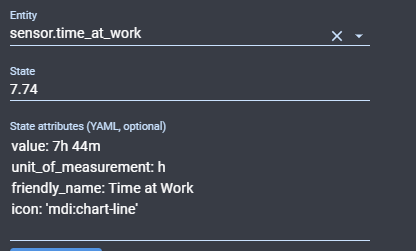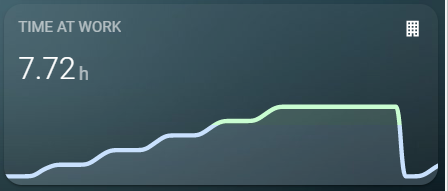# Create output from time sensor in hours and minutes instead of 7.43 hours

I have searched I promise, but a lot of the answers I didnt understand.

I have a graph, showing length of time at work.
It shows a time sensor in an hour format with a decimal place, ie it shows 7.43 hours, not 7hrs 25 minutes.

In states it shows the below:So it knows 7h 25m is the answer, but how can I get it to show that number here:You won’t be able to. You either display the time as time and get a bar graph, or you display the time as a base 10 number and get a graph.

You’re trying to mix some of a with some of b. You won’t be able to do this without something custom.

Thanks! How would I go about expressing the time as time though, that’s what is causing the main annoyance and sticking point, I just don’t understand how to take the decimal and make it a time.

You’ll need to make a template sensor that converts hours to hours:minutes

Use this template.

``````{% set hours = states('sensor.time_at_work') | float %}
{% set minutes = ((hours % 1) * 60) | int %}
{% set hours = (hours - (hours % 1)) | int %}
{{ '%02i:%02i'%(hours, minutes) }}
``````

`{% set hours = states('sensor.time_at_work') | float %}` - Gets your time at work, converts to float
`{% set minutes = ((hours % 1) * 60) | int %}` - Gets the remainder of hours / 1, then multiplies by 60 and converts to an int.
`{% set hours = (hours - (hours % 1)) | int %}` - Subtracts the remainder from hours and converts to an int
`{{ '%02i:%02i'%(hours, minutes) }}` - formats time so that it will be xx:xx for time (07:25 for 7.43). The minutes will truncate the remainder and 25.8 minutes will be 25.

Amazing thank you! Will give it a go once I work out why I can no longer connect to my Pi :s

With the new Entity card you can now specify “value” as an attribue so you can see proper time instead of decimal time. But it is still not possible with the graph card.

1 Like